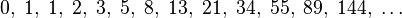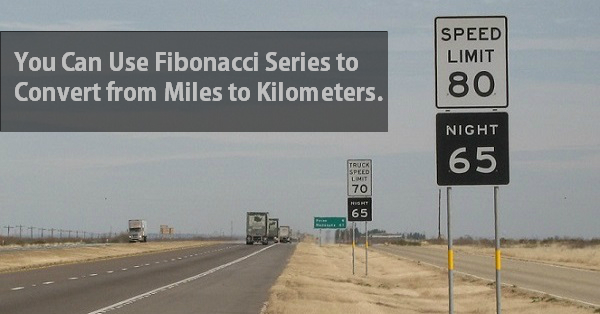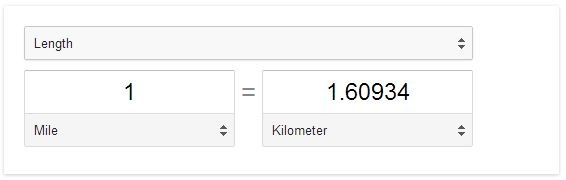## You Can Use Fibonacci Series to Convert from Miles to Kilometers

And you thought Fibonacci numbers is of no use?! A number is a Fibonacci series is (approximately) equivalent in kilometres to the previous number in miles allowing you to convert miles to kilometres and vice-versa in a ‘mathematical’ fashion.In mathematics, a Fibonacci series is made up numbers that are sum of the previous two numbers starting with 0 and 1.5 miles is 8 kilometres and 3 kilometres is 2 miles. Not only the Fibonacci series does the conversion for you, it also rounds off the result (except for the first two numbers in the series). For example 21 miles is exactly 33.7962 kilometres which rounds to 34 and similarly 144 kilometres is exactly 89.4775 in miles which rounds off to 89.### The Golden Ratio:

As we move towards higher numbers in Fibonacci series, the ratio of two consecutive numbers converges to 1.1618, also known as the golden ratio. The mile/kilometre ratio happens to be 1.6093 which is way close to this golden ratio hence can be used interchangeably if you’re not working on a space ship!

### But what is 100 Kilometers in Miles?

The series is very sparse with only thirteen values within 200 range but you can still convert 100 miles in kilometers using nothing but the Fibonacci series itself. Here’s how:
100 = 89 + 8 + 3
We don’t have 100 in the series but we do have an 89, a 8 and a 3, ahah!
100km = 89km + 8km + 3km
100km = 55m + 5m + 2m
100 kilometers = 62 miles (the exact answer is 62.1371)

This makes Fibonacci one less thing from your list of ‘math I learned in college with no use in real life’ 🙂

Edit: Or you can just type “100 km in miles” in Google and get the answer back.